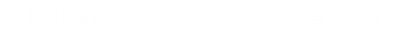Skip to main content# Rounding Invoices in Collaborati

This article describes how Collaborati handles rounding.

We implemented the unbiased rounding mechanism widely used in financials which is called half-even rounding (or convergent rounding, or Gaussian rounding).  This uses a tie-breaking rule in which half-way values are rounded toward the nearest even number.  For example, if you run your test with total = 70.115 – it will round it to 70.12, 70.125 will also round to 70.12, then 70.135 – will be rounded to 70.14, and 70.145 – will be also rounded to 70.14, etc. The idea is that the overall totals across all rounded values add up most closely to the rounded total.

Additionally, Collaborati will round all Rates, Hours, and Units to two decimal places before calculating the line item totals.  Collaborati will send Rates, Hours and Units to TeamConnect with two decimal places.  The Price for Expenses is limited by the number of decimals in the client’s TeamConnect settings.  This can be found on the Client’s Settings page in Collaborati.  If Non-US Taxes are used, they are also rounded to two decimal places.

In order to avoid any problems with rounding, we suggest using Round Rates (rounded to the nearest dollar) and also hours in tenths of an hour (such as 0.1 or 0.5).

Note that Collaborati also has an allowance rule:
In the INVOICE_TOTAL calculation, a 0.1% variable amount is allowed for numeric rounding and in the LINE_ITEM_TOTAL calculation, a 0.1% variable amount is allowed for numeric rounding.

• Was this article helpful?